Question

# Suppose that an airline uses a seat width of 16.5in. Assume men have hip breadths that...

Suppose that an airline uses a seat width of 16.5in. Assume men have hip breadths that are normally distributed with a mean of 14.7in. and a standard deviation of 1.1in. Complete parts​ (a) through​ (c) below.

​(a) Find the probability that if an individual man is randomly​ selected, his hip breadth will be greater than 16.5 in.The probability is ________

​(Round to four decimal places as​ needed.)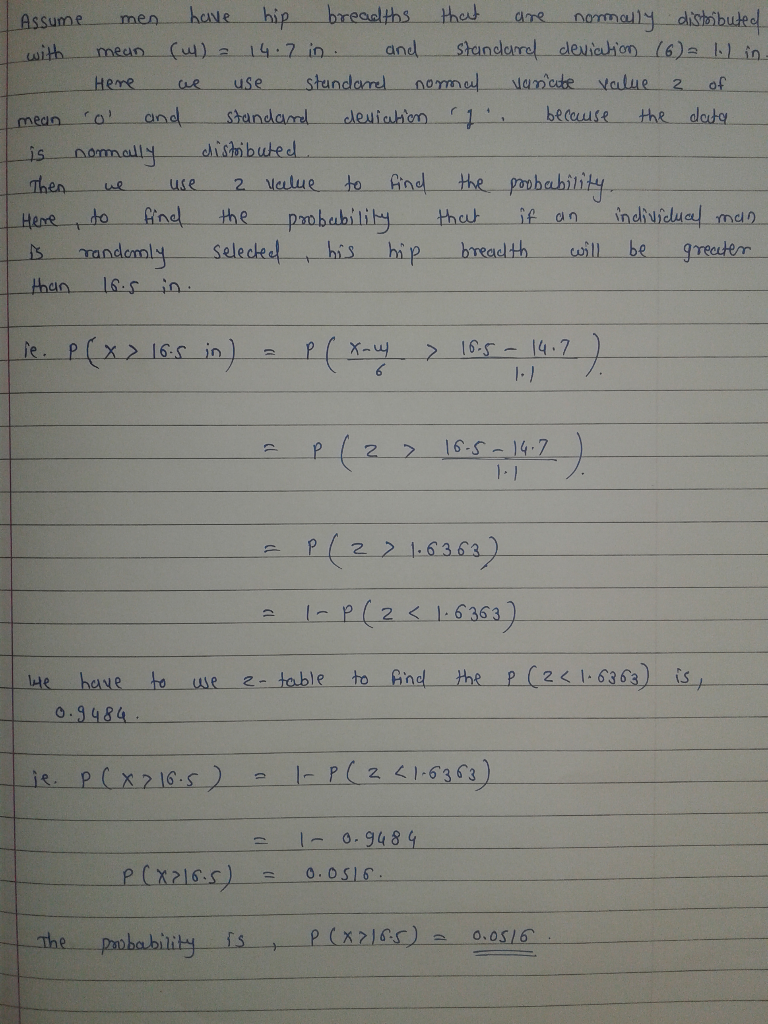#### Earn Coins

Coins can be redeemed for fabulous gifts.

Similar Homework Help Questions
• ### 5. Suppose that an airline uses a seat width of 16.3 in. Assume men have hip...

5. Suppose that an airline uses a seat width of 16.3 in. Assume men have hip breadths that are normally distributed with a mean of 14.4 in. and a standard deviation of 0.9 in. Complete parts​ (a) through​ (c) below. ​(a) Find the probability that if an individual man is randomly​ selected, his hip breadth will be greater than 16.3 in.The probability is nothing. ​(Round to four decimal places as​ needed.)

• ### Suppose that an airline uses a seat width of 16.8 in. Assume men have hip breadths...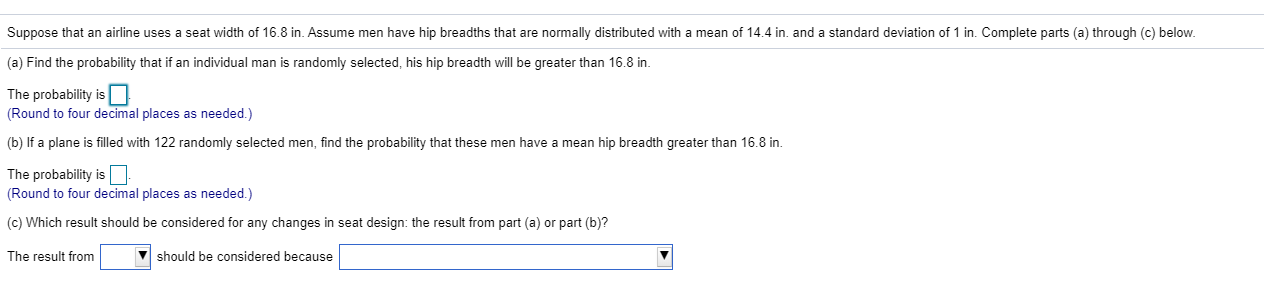Suppose that an airline uses a seat width of 16.8 in. Assume men have hip breadths that are normally distributed with a mean of 14.4 in, and a standard deviation of 1 in. Complete parts (a) through (c) below. (a) Find the probability that if an individual man is randomly selected, his hip breadth will be greater than 16.8 in. The probability is (Round to four decimal places as needed.) (b) If a plane is filled with 122 randomly selected...

• ### Suppose that an airline uses a seat width of 16.4 in. Assume men have hip breadths...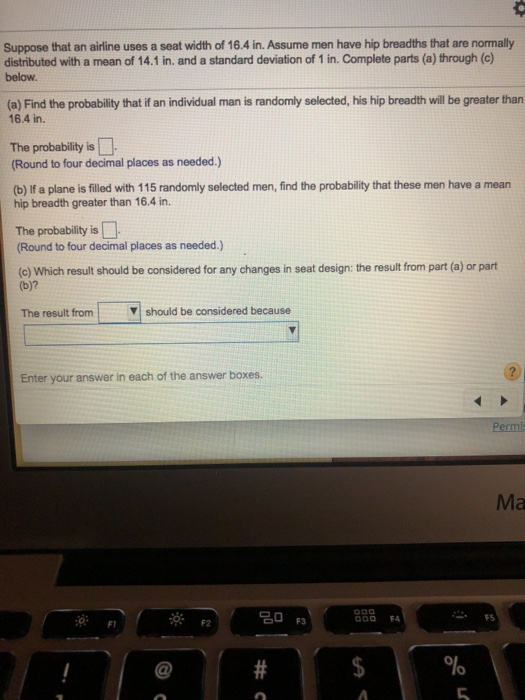Suppose that an airline uses a seat width of 16.4 in. Assume men have hip breadths that are normally distributed with a mean of 14.1 in, and a standard deviation of 1 in. Complete parts (a) through (c) below. (a) Find the probability that if an individual man is randomly selected, his hip breadth will be greater than 16.4 in. The probability is (Round to four decimal places as needed.) (b) If a plane is filled with 115 randomly selected...

• ### Suppose that an airline uses a seat width of 16.4 in. Assume men have hip breadths...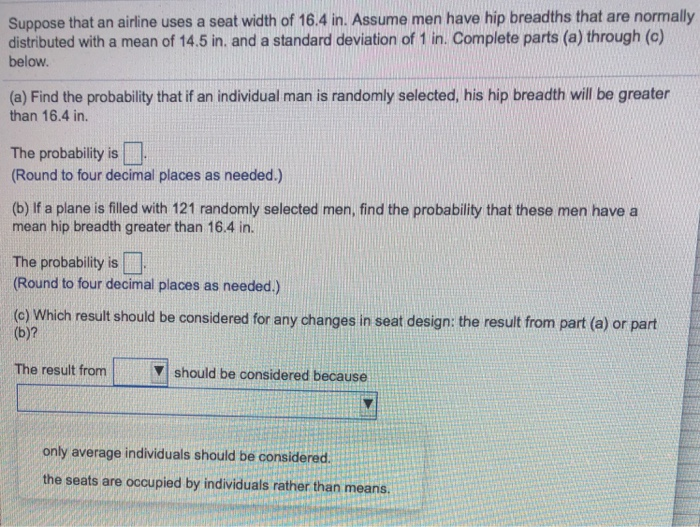Suppose that an airline uses a seat width of 16.4 in. Assume men have hip breadths that are normally distributed with a mean of 14.5 in. and a standard deviation of 1 in. Complete parts (a) through (c) below. (a) Find the probability that if an individual man is randomly selected, his hip breadth will be greater than 16.4 in. The probability is Round to four decimal places as needed.) (b) If a plane is filled with 121 randomly selected...

• ### Suppose that an airline uses a seat width of 16.7 in. Assume men have hip breadths that are normally distributed with a mean of 14.5 in.

Suppose that an airline uses a seat width of 16.7 in. Assume men have hip breadths that are normally distributed with a mean of 14.5 in. and a standard deviation of 1 in. Complete parts (a) through (c) below. (a) Find the probability that if an individual man is randomly selected, his hip breadth will be greater than 16.7 in. The probability is _______ (Round to four decimal places as needed.) (b) If a plane is filled with 114 randomly selected men, find...

• ### Suppose that an airline uses a seat width of 16.5 in. Assume men have hip breadthes...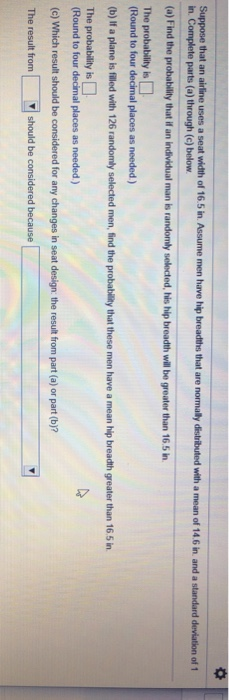Suppose that an airline uses a seat width of 16.5 in. Assume men have hip breadthes that are normally distributed with a mean of 14.6 in, and a standard deviation of 1 in Complete parts (a) through (c) below. (a) Find the probability that if an individual man is randomly selected, his hip breadth will be greater than 16.5 in. The probability is (Round to four decimal places as needed.) (b) If a plane is filled with 126 randomly selected...

• ### Suppose that an airline uses a seat width of 16.5 in. Assume men have hip breadths...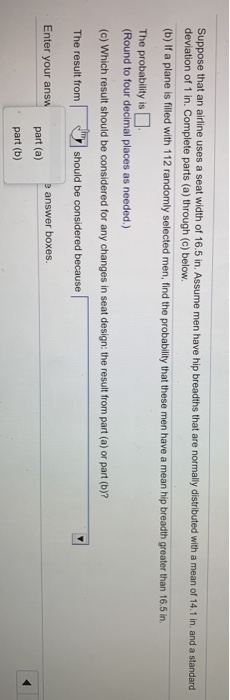Suppose that an airline uses a seat width of 16.5 in. Assume men have hip breadths that are normally distributed with a mean of 14.1 in and a standard deviation of 1 in. Complete parts (a) through (c) below. (b) If a plane is filled with 112 randomly selected men, find the probability that these men have a mean hip breadth greater than 16.5 in. The probability is a (Round to four decimal places as needed.) (c) Which result should...

• ### Suppose that an airline uses a seat width of 16 8 in Assume men have hip...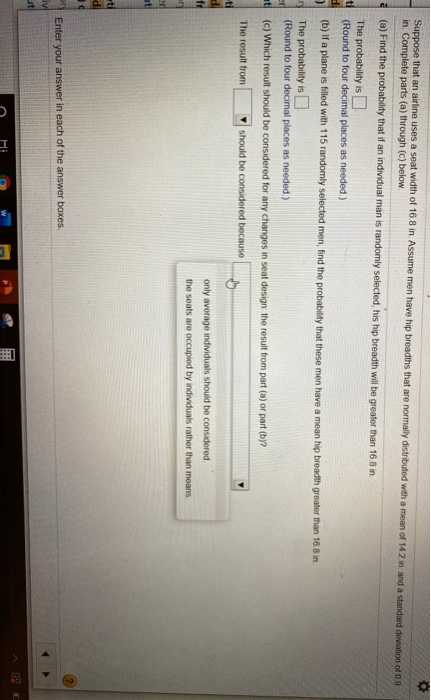Suppose that an airline uses a seat width of 16 8 in Assume men have hip breadths that are normally distributed with a mean of 142 in and a standard deviation of in Complete parts (a) through (c) below (a) Find the probability that if an individual man is randomly selected his hip breadth will be greater than 16 8 in The probability is (Round to four decimal places as needed) (b) If a plane is filled with 115 randomly...

• ### Suppose that an airline uses a seat width of 16.7 in. Assume men have hip breadths...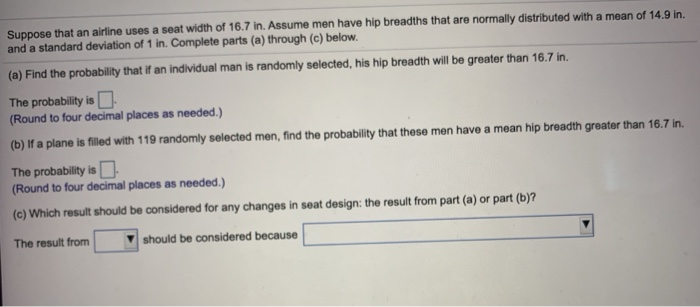Suppose that an airline uses a seat width of 16.7 in. Assume men have hip breadths that are normally distributed with a mean of 14.9 in. and a standard deviation of 1 in. Complete parts (a) through (c) below. (a) Find the probability that if an individual man is randomly selected, his hip breadth will be greater than 16.7 in. The probability is (Round to four decimal places as needed.) (b) If a plane is filled with 119 randomly selected...

• ### Suppose that an airline uses a seat width of 16.4 in

Suppose that an airline uses a seat width of 16.4 in. Assume men have hip breadths that are normally distributed with a mean of 14.5 in. and a standard deviation of 1.1 in. Complete parts (a) through (c) below (a) Find the probability that if an individual man is randomly selected, his hip breadth will be greater than 16.4 in. The probability is (Round to four decimal places as needed.) (b) If a plane is filled with 127 randomly selected men, find the...